# Reply to 'Mathematical Inconsistency in Solomonoff Induction?'

Date: 2020-09-04

This is a reply to this LessWrong post.

I went through the maths in OP and it seems to check out. I think the core inconsistency is that SI implies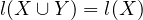. I’m going to redo the maths below (breaking it down step-by-step more). curi has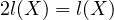which is the same inconsistency given his substitution. I’m not sure we can make that substitution but I also don’t think we need to.

Let X and Y be independent hypotheses for Solomonoff induction.

According to the prior, the non-normalized probability of X (and similarly for Y ) is: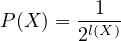(1)

what is the probability of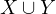?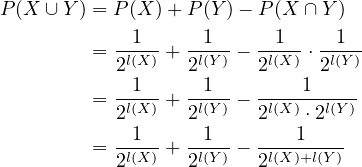(2)

However, by Equation (1) we have: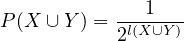(3)

thus(4)

This must hold for any and all X and Y .

curi considers the case where X and Y are the same length, starting with Equation (4)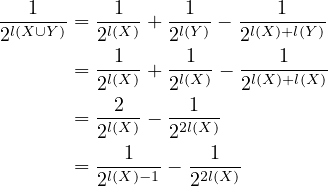(5)

but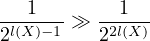(6)

and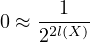(7)

so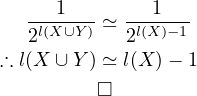(8)

curi has slightly different logic and argues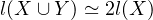which I think is reasonable. His argument means we get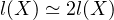. I don’t think those steps are necessary but they are worth mentioning as a difference. I think Equation (8) is enough.

I was curious about what happens when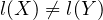. Let’s assume the following: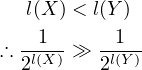(9)

so, from Equation (2)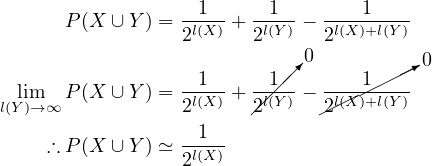(10)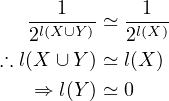(11)

but Equation (9) says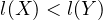– this contradicts Equation (11).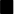So there’s an inconsistency regardless of whetheror not.